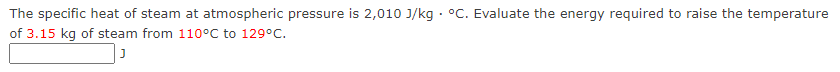Home / Expert Answers / Chemistry / q19-the-specific-heat-of-steam-at-atmospheric-pressure-is-2-010-mathrm-j-mathrm-kg-cdo-pa373

# (Solved): Q19 The specific heat of steam at atmospheric pressure is $$2,010 \mathrm{~J} / \mathrm{kg} \cdo ... Q19The specific heat of steam at atmospheric pressure is \( 2,010 \mathrm{~J} / \mathrm{kg} \cdot{ }^{\circ} \mathrm{C}$$. Evaluate the energy required to raise the temperature of $$3.15 \mathrm{kq}$$ of steam from $$110^{\circ} \mathrm{C}$$ to $$129^{\circ} \mathrm{C}$$. $\text { J }$

We have an Answer from Expert